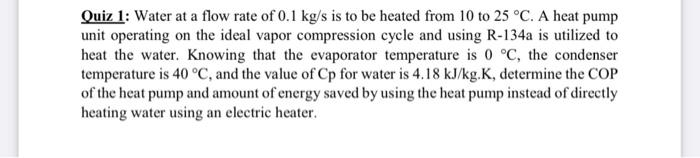# Question Solved1 Answerineed very fast answer in 20 min Quiz 1: Water at a flow rate of 0.1 kg/s is to be heated from 10 to 25 °C. A heat pump unit operating on the ideal vapor compression cycle and using R-134a is utilized to heat the water. Knowing that the evaporator temperature is 0 °C, the condenser temperature is 40 °C, and the value of Cp for water is 4.18 kJ/kg.K, determine the COP of the heat pump and amount of energy saved by using the heat pump instead of directly heating water using an electric heater.4IJ5PV The Asker · Mechanical Engineering
ineed very fast answer in 20 minTranscribed Image Text: Quiz 1: Water at a flow rate of 0.1 kg/s is to be heated from 10 to 25 °C. A heat pump unit operating on the ideal vapor compression cycle and using R-134a is utilized to heat the water. Knowing that the evaporator temperature is 0 °C, the condenser temperature is 40 °C, and the value of Cp for water is 4.18 kJ/kg.K, determine the COP of the heat pump and amount of energy saved by using the heat pump instead of directly heating water using an electric heater.
More
Transcribed Image Text: Quiz 1: Water at a flow rate of 0.1 kg/s is to be heated from 10 to 25 °C. A heat pump unit operating on the ideal vapor compression cycle and using R-134a is utilized to heat the water. Knowing that the evaporator temperature is 0 °C, the condenser temperature is 40 °C, and the value of Cp for water is 4.18 kJ/kg.K, determine the COP of the heat pump and amount of energy saved by using the heat pump instead of directly heating water using an electric heater.